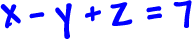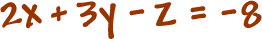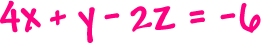Before, we learned how to solve systems with two equations and two unknowns...  2 x 2's.

Here's an example of the type we solved: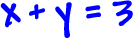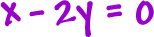Remember that these are two lines: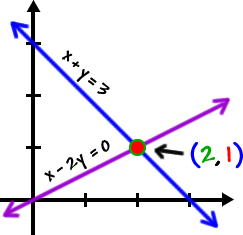The point where the two lines intersect is the solution: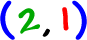So, x = 2 and y = 1 work in BOTH equations.

Now, we're going to do 3 x 3's -- systems with three equations and three unknowns.

Like this guy: